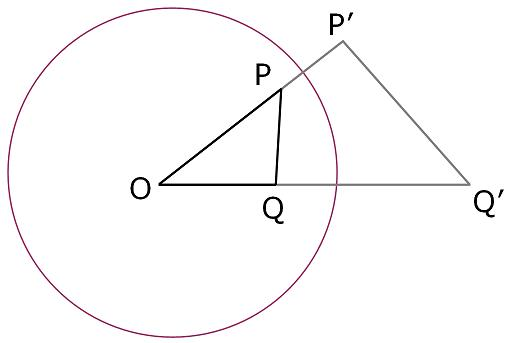#### You may also like### Fitting In

The largest square which fits into a circle is ABCD and EFGH is a square with G and H on the line CD and E and F on the circumference of the circle. Show that AB = 5EF. Similarly the largest equilateral triangle which fits into a circle is LMN and PQR is an equilateral triangle with P and Q on the line LM and R on the circumference of the circle. Show that LM = 3PQ### Look Before You Leap

Can you spot a cunning way to work out the missing length?### Triangle Midpoints

You are only given the three midpoints of the sides of a triangle. How can you construct the original triangle?

# Points in Pairs

##### Age 14 to 16Challenge Level

We had a couple of correct solutions (500/48 or 10.4 to 3sf) using the Cosine Rule, and one solution which used the much simpler method of similar triangles:I noticed that, since $OP'=100/OP$ and $OQ'=100/OQ$, the ratio $\frac{OP}{OQ}$ is $\frac{OQ'}{OP'}$ and so the triangles $OPQ$ and $OQ'P'$ are similar.

So, in this question $OP'=100/8$ and, since the ratio between lengths of sides is the same by similar triangles, $$\frac{P'Q'}{OP'}=\frac{PQ}{OQ}.$$ Therefore, $$P'Q'=OP'\times\frac{PQ}{OQ}=\frac{100}{8}\times\frac{5}{6}=\frac{500}{48}.$$.